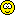Original Post
Junior Member
Black Belt
Join Date: Apr 2011
Posts: 13
Hello everyone! ^.^
My name is Retrou and I love to bet. I made an application to help myself with calculating earnings from bets.

this app can show you:
-House rake
-Winner earnings
-Most profitable bet
-When you reach max profit

Unfortunately you have to write the total ammount of bets, the app is very primitive itself.

You can download it freeware, I hope somebody will find this application usefullSource code code:
``````int main()
{

int a = 0;
for (int i = 1; i == 1; i++)
{
cout << "Welcome to TC bet calculator. \n";
if (a == 0)
{
double  x2, y1, y2;
cout << "1st player total bet TC ammount:";
cin >> y1;
cout << "2nd player total bet TC ammount:";
cin >> y2;
cout << "\t\t\t1st player wins:\n";
cout << "House rake/winner earnings:\t\t" << 0.1 * y2;
cout << "\nBets:\t\t\t";
for (int c = 100; c < 1000; c = c + 100)
{
if ((0.8*y2)*(c / (y1 + c)) >= 5 * c)
cout << "\n" << c << ":\t\t\t\t\t" << c*5 << "\treached max profit";
else
cout << "\n" << c << ":\t\t\t\t\t" << (0.8*y2)*(c / (y1 + c));
}
for (int d = 1000; d < 5000; d = d + 1000)
{
if ((0.8*y2)*(d / (y1 + d)) >= 5 * d)
cout << "\n" << d << ":\t\t\t\t\t" << d * 5 << "\treached max profit";
else
cout << "\n" << d << ":\t\t\t\t\t" << (0.8*y2)*(d / (y1 + d));
}
for (int j = 5000; j < 105000; j = j + 5000)
{
if ((0.8*y2)*(j / (y1 + j)) >= 5 * j)
cout << "\n" << j << ":\t\t\t\t\t" << j * 5 << "\treached max profit";
else
cout << "\n" << j << ":\t\t\t\t\t" << (0.8*y2)*(j / (y1 + j));
}
cout << "\n\t\t\t2nd player wins:\n";
cout << "House rake/winner earnings:\t\t" << 0.1 * y1;
cout << "\nBets:\t\t\t";
for (int q = 100; q < 1000; q = q + 100)
{
if ((0.8*y1)*(q / (y2 + q)) >= 5 * q)
cout << "\n" << q << ":\t\t\t\t\t" << q * 5 << "\treached max profit";
else
cout << "\n" << q << ":\t\t\t\t\t" << (0.8*y1)*(q / (y2 + q));
}
for (int w = 1000; w < 5000; w = w + 1000)
{
if ((0.8*y1)*(w / (y2 + w)) >= 5 * w)
cout << "\n" << w << ":\t\t\t\t\t" << w * 5 << "\treached max profit";
else
cout << "\n" << w << ":\t\t\t\t\t" << (0.8*y1)*(w / (y2 + w));
}
for (int r = 5000; r < 105000; r = r + 5000)
{
if ((0.8*y1)*(r / (y2 + r)) >= 5 * r)
cout << "\n" << r << ":\t\t\t\t\t" << r * 5 << "\treached max profit";
else
cout << "\n" << r << ":\t\t\t\t\t" << (0.8*y1)*(r / (y2 + r));
}
cout << "\n\ndo you want to proceed? (type 0 to continue)\n:";
cin >> i;
}
else;
}

return 0;
}``````

If you have some questions or suggestions for the app, please make a comment. I will try to do my best!

If you want to support me or my content, send some TCSee ya in the betting room!

p.s. dont worry its not trojan.
p.p.s. dont laugh at the code, im a begginer.
Last edited by Retrou; Mar 27, 2018 at 11:24 PM. Reason: adding source code